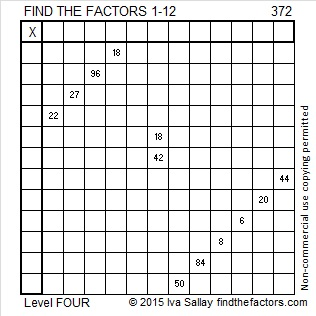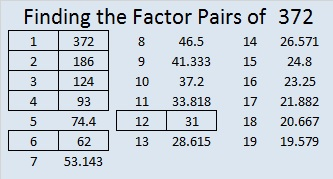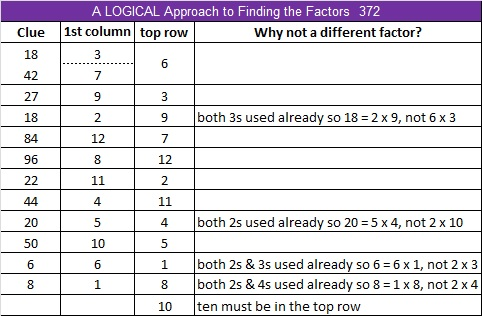# 372 and Level 4

372 is even and therefore a composite number. Scroll down for its factoring information.Print the puzzles or type the factors on this excel file:12 Factors 2015-01-26

• 372 is a composite number.
• Prime factorization: 372 = 2 x 2 x 3 x 31, which can be written 372 = (2^2) x 3 x 31
• The exponents in the prime factorization are 2, 1, and 1. Adding one to each and multiplying we get (2 + 1)(1 + 1)(1 + 1) = 3 x 2 x 2 = 12. Therefore 372 has exactly 12 factors.
• Factors of 372: 1, 2, 3, 4, 6, 12, 31, 62, 93, 124, 186, 372
• Factor pairs: 372 = 1 x 372, 2 x 186, 3 x 124, 4 x 93, 6 x 62, or 12 x 31
• Taking the factor pair with the largest square number factor, we get √372 = (√4)(√93) = 2√93 ≈ 19.287This site uses Akismet to reduce spam. Learn how your comment data is processed.